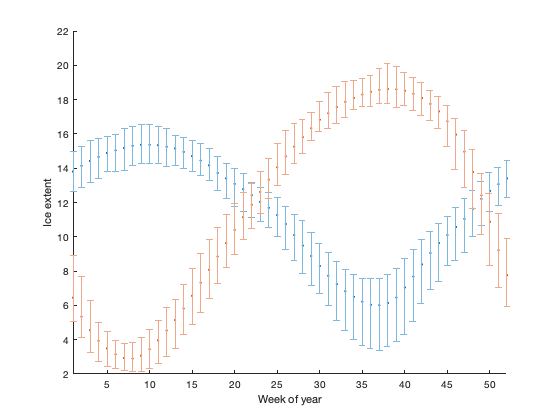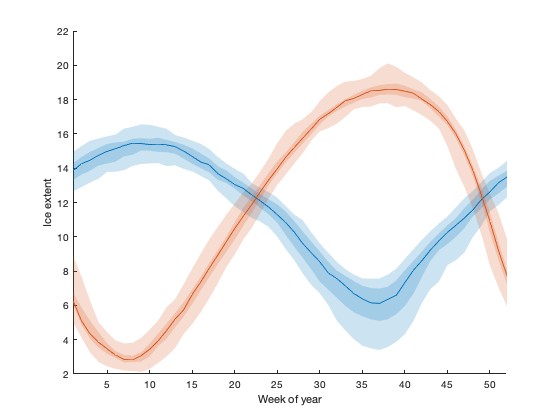# ensemble2bnd documentation

The ensemble2bnd function calculates statistics across an ensemble of datasets, and provides several options to plot these ensemble statistics.

In this context, we use "ensemble" to refer to any set of vector datasets using the same independent-variable coordinates. Ensemble data is common in climate science; model studies often use intra-model ensembles to encompass uncertainty in initial conditions or parameters, and intermodel ensembles to further encompass structural uncertainties in model processes. Timeseries data is also a prime candidate for this type of analysis, since we often want to look at interannual variability.

The ensemble2bnd function was originally created to feed ensemble data into the boundedline function, and has expanded to allow several options to plot the mean, median, quartiles, upper/lower bounds, etc. of these types of datasets.

Back to Climate Data Tools Contents

## Syntax

```A = ensemble2bnd(x,y)
[A, h] = ensemble2bnd(x,y, 'plot', plottype)
[...] = ensemble2bnd(..., 'dims', dims)
[...] = ensemble2bnd(..., 'center', center)
[...] = ensemble2bnd(..., 'prc', prc)
[...] = ensemble2bnd(..., 'alpha', alphaval)
[...] = ensemble2bnd(..., 'tlim', tlim)
[...] = ensemble2bnd(..., 'cmap', colors)
[...] = ensemble2bnd(..., 'axis', hax)
[...] = ensemble2bnd(..., 'whisker', w)```

## Description

[A, h] = ensemble2bnd(x,y) calculates the mean and upper/lower bounds of y at each x-coordinate. The x data should be a vector array, and y is a nx x ny x nens array, where nx corresponds to the number of x-dimension points (i.e. length of x input), ny corresponds to the number of different datasets, and nens is the number of ensemble members in each dataset. (See 'dims' parameter to permute these dimensions)

[A, h] = ensemble2bnd(x,y, 'plot', plottype) plots the datasets as either lines with shaded patches indicating percentiles ('boundedline'), lines with errorbars ('errorbar'), unstacked bar plots with errorbars ('bar'), or boxplots ('boxplot'). The default, 'none', indicates no plots will be created.

ensemble2bnd(..., 'dims', dims) allows for permutation of the input matrix y via a 3-letter string consisting of the letters x, y, and e that indicate which dimensions in the input matrix correspond to the independent coordinate, the different datasets, and the ensembles per dataset, respectively. The default is 'xye'.

ensemble2bnd(..., 'center', center) switches the center-line statistic between the 'mean' (default) and 'median' of each dataset.

ensemble2bnd(..., 'prc', prc) specifies the percentile values corresponding to lower and upper bounds. These should come in pairs, from lowest to highest percentile. The default is [0 100].

ensemble2bnd(..., 'alpha', true) indicates that boundedline patches should be plotted using tranparent patches rather than opaque patches.

ensemble2bnd(..., 'tlim', tlim) changes the color limits associated with the patches or errorbars associated with percentiles. By default, the center lines/bars/points are drawn with the indicated color (saturation = 1), and colors of bounds objects (patch, errorbars, etc) use lighter versions of the color, getting lighter as bounds expand (default limits are [0.1 0.5]).

ensemble2bnd(..., 'cmap', colors) provides an alternative color order for the datasets plotted.

ensemble2bnd(..., 'axis', hax) adds any plots to the indicated axis rather than the current axis.

ensemble2bnd(..., 'whisker', w) changes te whisker length factor used to calculated outliers for the boxplot (boxplot option only).

## Example: Sea ice interannual variability

For our example, we'll use the sea ice extent timeseries as our ensemble data. We'll start by reshaping the two timeseries to year x time-of-year matrices using reshapetimeseries, and concatenating these. The early data uses a two-day interval, while the later is daily; we'll bin data weekly to avoid any artifacts from this change:

```load seaice_extent.mat

iceN = reshapetimeseries(t, extent_N, 'bin', 52);
iceS = reshapetimeseries(t, extent_S, 'bin', 52);

ice = cat(3, iceN, iceS);
```

By default, the function calculates the mean and upper/lower bounds of the data at each x-location in the data. We can plot either bounded lines:

```[A, h] = ensemble2bnd(1:52,ice, 'dims', 'xey', 'plot', 'boundedline')
set(gca,'xlim', [1 52]);
xlabel('Week of year');
ylabel('Ice extent');
```
```A =
struct with fields:

cent: [52×2 double]
bndlo: [52×2 double]
bndhi: [52×2 double]
errlo: [52×2 double]
errhi: [52×2 double]
h =
struct with fields:

ln: [1×2 Line]
patch: [1×2 Patch]
```line plots with error bars:

```cla
[A, h] = ensemble2bnd(1:52,ice, 'dims', 'xey', 'plot', 'errorbar')
```
```A =
struct with fields:

cent: [52×2 double]
bndlo: [52×2 double]
bndhi: [52×2 double]
errlo: [52×2 double]
errhi: [52×2 double]
h =
struct with fields:

ln: [2×1 Line]
errbar: [1×2 ErrorBar]
```or bar plots with errorbars:

```cla
[A,h] = ensemble2bnd(1:52, ice, 'dims', 'xey', 'plot', 'bar')
set(h.bar, 'edgecolor', 'none');
```
```A =
struct with fields:

cent: [52×2 double]
bndlo: [52×2 double]
bndhi: [52×2 double]
errlo: [52×2 double]
errhi: [52×2 double]
h =
struct with fields:

bar: [1×2 Bar]
errbar: [1×2 ErrorBar]
```Note that the field names in the handle output structures vary based upon the plotting option you choose.

We can also increase the number of intervals shown. For example, perhaps you'd like to see interquartile ranges as well as the minimum and maximum. In this case, it may make more sense to use the median as the center line rather than the mean:

```cla
ensemble2bnd(1:52,ice, 'dims', 'xey', 'plot', 'boundedline', ...
'cent', 'median', 'prc', [0 25 75 100]);
```If you add a large number of evenly-spaced percentiles, you can mimic a shaded distribution plot.

```cla
[~,h] = ensemble2bnd(1:52,ice, 'dims', 'xey', 'plot', 'boundedline', ...
'cent', 'median', 'prc', [0:2:48 52:2:100], 'tlim', [0.1 0.9]);
```The plotting order of the patch objects moves from outer bounds to inner bounds, which is what leads to the interleaved appearance where the lines overlap, but you can fix that with a bit of restacking:

```uistack(h.patch(end:-1:1,2), 'top');
```As an alternative to the opaque bounds with varying colors, you can plot the bounds using transparent patches. When using transparent patches, it often makes sense to set the transparency limits to a single value, since overlapping patches will automatically show an additive color:

```cla
[~,h] = ensemble2bnd(1:52,ice, 'dims', 'xey', 'plot', 'boundedline', ...
'cent', 'median', 'prc', [0 25 75 100], 'tlim', [0.2 0.2], ...
'alpha', true);
```The boxplot plotting option works a little differently than the other options, in that you can't specify the exact percentile bounds to plot. Instead, this option uses the usual boxplot statistics (note that this option requires the Statistics Toolbox, since it uses the boxplot function from that toolbox). Like the bar plot option, this version places datasets side-by-side to avoid too much overlap.

The graphics handles returned by the boxplot function are... unintuitive, to say the least. If you want to change or edit properties, I recommend using tags:

```cla
[A,h] = ensemble2bnd(1:52,ice, 'dims', 'xey', 'plot', 'boxplot')

set(findall(h.box, 'tag', 'Upper Whisker'), 'linestyle', '-');
set(findall(h.box, 'tag', 'Lower Whisker'), 'linestyle', '-');
```
```A =
struct with fields:

cent: [52×2 double]
bndlo: [52×2×2 double]
bndhi: [52×2×2 double]
errlo: [52×2×2 double]
errhi: [52×2×2 double]
h =
struct with fields:

box: [7×2×52 double]
```## Author Info

The ensemble2bnd function and its supporting documenation were written by Kelly A. Kearney of the University of Washington. The ensemble2bnd function is part of the Climate Data Toolbox for Matlab.